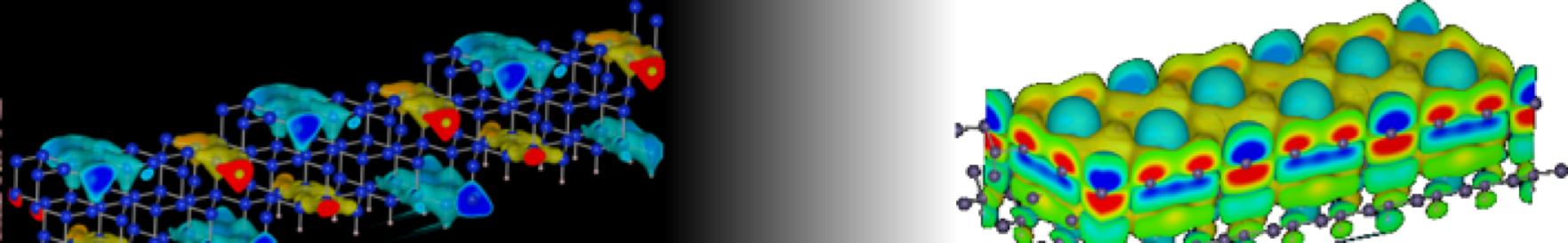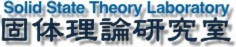SST Lab Dokuwiki Header#@surface

### サイドバー

SST Lab Dokuwiki Headerase:cutoff

# エネルギーカットオフ

## set_pw

set_pw(self, pw)

set the planewave cutoff.

### Parameters

 pw integer the planewave cutoff in eV

this function checks to make sure the density wave cutoff is greater than or equal to the planewave cutoff.

## set_dw

set_dw(self, dw)

set the density wave cutoff energy.

### Parameters

 dw integer the density wave cutoff

The function checks to make sure it is not less than the planewave cutoff.

Density_WaveCutoff, $E_{cut}$, describes the kinetic energy necessary to represent a wavefunction associated with the total density, i.e. $\vec{G}$-vectors for which $|\vec{G}|^2<4E_{cut}$ will be used to describe the total density (including augmentation charge and partial core density). If Density_WaveCutoff is equal to PlaneWaveCutoff this implies that the total density is as soft as the wavefunctions described by the kinetic energy cutoff PlaneWaveCutoff. If a value of Density_WaveCutoff is specified (must be larger than or equal to PlaneWaveCutoff) the program will run using two grids, one for representing the wavefunction density (softgrid_dim) and one representing the total density (hardgrid_dim). If the density can be reprensented on the same grid as the wavefunction density Density_WaveCutoff can be chosen equal to PlaneWaveCutoff (default).

## get_pw

get_pw(self)

return the planewave cutoff used

print jacapo.get_pw()

## get_dw

get_dw(self)

return the density wave cutoff

ase/cutoff.txt · 最終更新: 2019/05/21 19:32 by kimi

### ページ用ツール